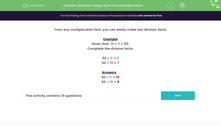# Find the Related Division Facts

In this worksheet, students will learn to write down two simple division facts from a multiplication fact.Key stage:  KS 2

Curriculum topic:   Number: Multiplication and Division

Curriculum subtopic:   Write Multiplication/Division Statements for Known Tables

Popular topics:   Multiplication worksheets, Division worksheets

Difficulty level:#### Worksheet Overview

In this activity, we will be exploring the connection between multiplication and division facts.

From any multiplication fact, you can easily make two division facts.

We know that

10 × 5 = 50

Fifty contains ten lots of five or five lots of ten.

From this multiplication fact, we can write two related division facts.

50 ÷ 10 = 5

50 ÷ 5 = 10

We can see that the same numbers have been used but rearranged from the multiplication fact.

Let's try an example question together.

Example

3 × 5 = 15

Complete the division facts.

15 ÷ 5 = ?

15 ÷ 3 = ?

To complete the division facts, we simply check for the missing number from the multiplication fact.

3 × 5 = 15

15 ÷ 5 = 3

15 ÷ 3 = 5

Now it's your turn to try some questions like this.### What is EdPlace?

We're your National Curriculum aligned online education content provider helping each child succeed in English, maths and science from year 1 to GCSE. With an EdPlace account you’ll be able to track and measure progress, helping each child achieve their best. We build confidence and attainment by personalising each child’s learning at a level that suits them.

Get started